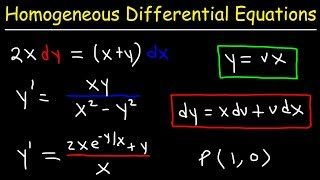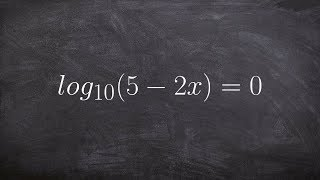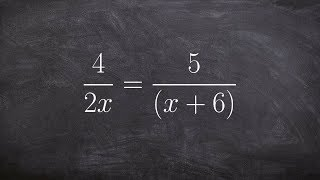Try NerdPal: our new app on iOS and Android !

Related Videos

Solve the differential equation $\frac{dy}{dx}=\frac{2x}{3y^2}$

Go!
Go!
1
2
3
4
5
6
7
8
9
0
a
b
c
d
f
g
m
n
u
v
w
x
y
z
.
(◻)
+
-
×
◻/◻
/
÷
2

e
π
ln
log
log
lim
d/dx
Dx
|◻|
θ
=
>
<
>=
<=
sin
cos
tan
cot
sec
csc

asin
acos
atan
acot
asec
acsc

sinh
cosh
tanh
coth
sech
csch

asinh
acosh
atanh
acoth
asech
acsch

VideosHomogeneous Differential EquationsAlgebra: Linear equations 2 | Linear equations | Algebra I | Khan AcademyTutorial - Solving logarithmic equations ex 5, log(5-2x)=0Creating a slope field | First order differential equations | Khan AcademyTutorial - Solving a rational Equation ex 6, 4/2x = 5/(x+6)SnapXam A2

beta Got another answer? Verify it!

Go!
1
2
3
4
5
6
7
8
9
0
a
b
c
d
f
g
m
n
u
v
w
x
y
z
.
(◻)
+
-
×
◻/◻
/
÷
2

e
π
ln
log
log
lim
d/dx
Dx
|◻|
θ
=
>
<
>=
<=
sin
cos
tan
cot
sec
csc

asin
acos
atan
acot
asec
acsc

sinh
cosh
tanh
coth
sech
csch

asinh
acosh
atanh
acoth
asech
acsch

$\frac{dy}{dx}=\frac{2x}{3y^2}$